The coefficient of performance of a refrigerator is 5. If the temperature inside freezer is -20°C, the temperature of the surroundings to which it rejects heat is  -

(a)31°C

(b)41°C

(c)11°C

(d)21°C

Concept Questions :-

Heat engine and refrigerator
High Yielding Test Series + Question Bank - NEET 2020

Difficulty Level:

A thermodynamic system undergoes cyclic process ABCDA as shown in figure. The work done by the system in the cycle is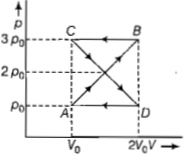(a) ρoV

(b) 2ρoVo

(c) ρoVo/2

(d)zero

Concept Questions :-

Cyclic process
High Yielding Test Series + Question Bank - NEET 2020

Difficulty Level:

A gas is taken through the cycle A→B→C→A, as shown. What is the net work done by the gas?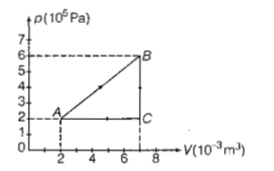(a)2000J

(b)1000J

(c)Zero

(d)-2000J

Concept Questions :-

Work done by gas
High Yielding Test Series + Question Bank - NEET 2020

Difficulty Level:

During an adiabatic process. the pressure of a gas is found to be proportional to the cube of its
temperature. The ratio of CP/CV for the gas is

(a) 4/3

(b) 2

(c) 5/3

(d) 3/2

Concept Questions :-

Molar Specific heat
High Yielding Test Series + Question Bank - NEET 2020

Difficulty Level:

A thermodynamic system is taken through the cycle ABCD as shown in figure. Heat rejected by the gas during the cycle is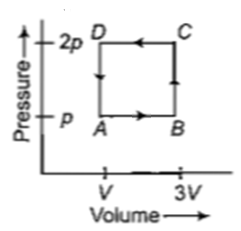(a)2 pV                                     (b)4 pV

(c)$\frac{1}{2}pV$                                    (d)pV

Concept Questions :-

First law of thermodynamics
High Yielding Test Series + Question Bank - NEET 2020

Difficulty Level:

One mole of an ideal gas from an initial state B undergoes via two processes. It first undergoes isothermal expansion from volume V to 3V and then its volume is reduced from 3V to V at constant pressure. The correct p-V diagram representing the two processes is -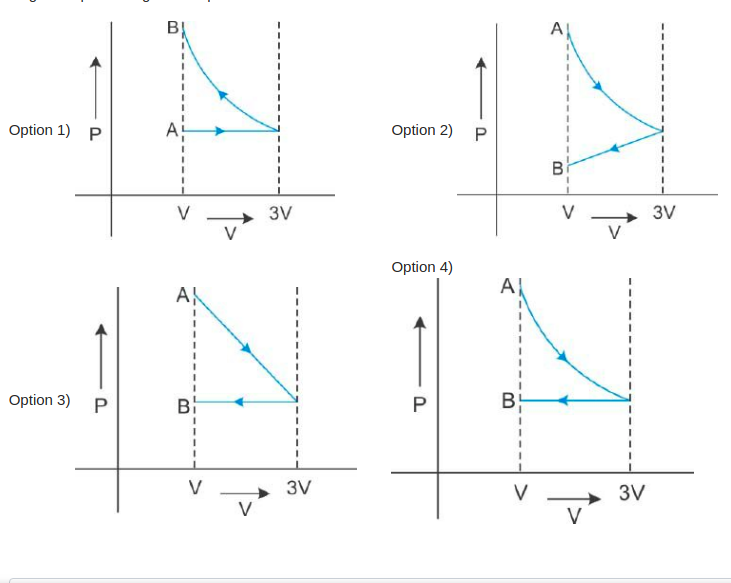Concept Questions :-

Types of processes
High Yielding Test Series + Question Bank - NEET 2020

Difficulty Level:

An ideal gas goes from state A to state B

via three different processes as indicated

in the p-V diagram

If  indicates the heat absorbed

by the gas along the three processes and

indicates the change in

internal energy along the three processes

respectively, then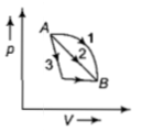(a)

(b)

(c)

(d)

Concept Questions :-

First law of thermodynamics
High Yielding Test Series + Question Bank - NEET 2020

Difficulty Level:

A mass of diatomic gas ($\gamma$=1.4) at a pressure of 2 atm is compressed adiabatically so that its temperature rise from $27°\mathrm{C}$ to $927°\mathrm{C}.$ The pressure of the gas is final state is-

(a) 28 atm                                            (b) 68.7 atm

(c) 256 atm                                           (d) 8 atm

Concept Questions :-

Types of processes
High Yielding Test Series + Question Bank - NEET 2020

Difficulty Level:

If  represent the increase in internal energy and work done by the system respectively in a thermodynamical process,which of the following is true?

(a) $∆U=-∆W,$ in a adiabatic process

(b) $∆U=∆W,$ in a isothermal process

(c) $∆U=∆W,$ in adiabatic process

(d) $∆U=-∆W,$in a isothermal process

Concept Questions :-

Types of processes
High Yielding Test Series + Question Bank - NEET 2020

Difficulty Level:

A monoatomic gas at pressure ${\mathrm{p}}_{1}$ and ${\mathrm{V}}_{1}$ is compressed adiabatically to $\frac{1}{8}\mathrm{th}$ its original volume. What is the final pressure of the gas ?

(a)                                    (b) ${\mathrm{p}}_{1}$

(c)                                     (d)

Concept Questions :-

Types of processes